## Mathematics Paper 2 Questions and Answers - Form 3 Mid Term 2 Exams 2021

Instructions

• Answer all the questions in section A and ONLY Five in section B.
• Show all the calculations in the spaces provided
• KNEC mathematical tables and non-programmable calculators may be used.

SECTION A

1. Use logarithm tables to evaluate; [4 Marks]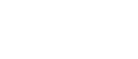2. A number is such that when it is divided by 3,7,11 or 13, the remainder is always 1. Find the number. [2 Marks]
3. A square has an area of 144m2. Calculate its perimeter. [2 Marks]
4. Factorise  2x2 − x − 6 hence solve the quadratic equation [3 Marks]
2x2 − x − 6 = 0
5. List all integral values of that satisfy the combined inequality; Represent the solutions on a single number line. [4 Marks]
6. A body accelerates at 5m/sto reach a velocity of 60m/s in 5 seconds. Calculate the initial velocity.   [2 Marks]
7.                                                                                        →     →      →
Draw a triangle STR and put arrows on its side to show that TS + SR = TR [ 2 Marks]
8. A point P(2,5) is translated to P’(1,6)
1. Find the translation vector [2 Marks]
2. The image of (3,0) under the same translation. [2 Marks]
9. Solve for in [3 Marks]
10. The sum of interior angles of a regular polygon is 3240°. Find the size of each exterior angle.  [3 Marks]
11. Write 1936 and 1728 in terms of its prime factors hence evaluate;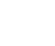12. Use reciprocal tables to evaluate; [4 Marks].
16    +     24
2.674     0.1396
13. Evaluate; [3 Marks]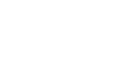14. Use substitution method to solve; (3 Marks)
2x + 3y = 1
3x − 2y = 8
15. The straight line joining the points P(a,7) and Q(13,a) is parallel to the line whose equation is 3y+2x=9. Find the value of a. [3 Marks]
16. The ratio of the areas of two circles is 16:25
1. What is the ratio of their radii. [2 Marks]
2. If the smaller circle has a diameter of 28cm, find the radius of the larger circle.
[2 Marks]

SECTION B

1. The marks of 30 girls in a class were recorded as follows.
220 250 204 230 210 227 221 252
200 228 208 225 200 202 240 228
212 225 252 216 212 226 227
240 248 203 201 251 242 216
1. Construct a frequency table with a class width of 5 Marks beginning with 199 marks. [3 Marks]
2. What is the modal class? [1 Mark]
3. Estimate the mean [3 Marks]
4. Estimate the median [3 Marks]
2. The initial velocity of a body is 30m/s. the body accelerates uniformly to a velocity of 60m/s in 6seconds. It moves at this constant velocity for 5 seconds before decelerating in 3 seconds.
1. Using the graph paper provided, draw a velocity time graph to illustrate the information above.   [4 Marks]
2. Calculate the initial acceleration [2 Marks]
3. Calculate the total distance covered. [4 Marks]
3. The diagram below shows two circles that share a common chord XY which is 13cm long.
Calculate;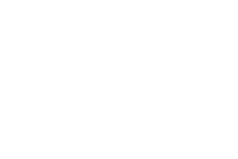1. <XO1Y [1 Mark]
2. < XO2Y[1 Mark]
3. The area of the sector O1XBY [2 Marks]
4. The area of the sector O2YAX [2 Marks]
5. The area of the shaded part [4 Marks]
4.
1. The diagram below shows a triangle OAB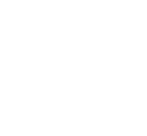Points M and K are on AB and OA respectively such that;
AM:MB=2:3 and K is the mid point of OA.
Express the following vectors interms of a and b.
1.  →
AB [1 Mark]
2.  →
OM [2 Marks]

3. BK [2 Marks]
2. The co-ordinates of P and Q are (6,10) and (8,14) respectively. Calculate;

1. PQ [1 Mark]
2. The mid-point of line PQ [2 Marks]
3. Given that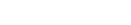. Another vector P is such that p=2a − b + 4c
Evaluate /P/ correct to 2 decimal places. [2 Marks]
5. The diagram below shows a frustrum that was cut from a right cone.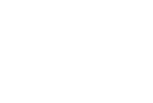Calculate;
1. The highest of the cone [2 Marks]
2. The volume of the frustrum [4 Marks]
3. The surface area of the frustrum [4 Marks]
6. A line L1 has the equation 3x + 4y = 12
1. Calculate
1. The gradient of line L1[2 Marks]
2. The coordinates of P and Q where the line cuts the x-axis and y-axis respectively [4 Marks]
3. Another line L2 is perpendicular to L1 and passes through (-4,5). Determine the equation of line L2 in the form [4 Marks]
7. Form all inequalities that define Region R [10 Marks]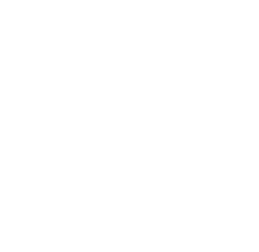8.
1. Two trains T1 and T2 travelling in opposite directions on parallel trucks are just beginning to pass each other. Train T1 is 72m long and is travelling at 108km/hr and T2 is 78m long travelling at 72km/hr. Find the time in seconds it takes the two trains take to completely pass one another.    [3 Marks]
2. A rally car travelled for 2 hours 40 minutes at an average speed of 120km/hr. the car consumes an average of 1 litre of fuel for every 4 kilometres.
A litre of fuel costs sh. 64. Calculate the amount of money spent on the fuel.
[4 Marks]
3. Mwangi and Otieno live 40km apart. Mwangi starts from his home at 7.30am and travels towards Otieno at 16km/hr. Otieno starts from his home at 8.00am and cycles at 8km/hr towards mwangi. At what time do they meet? [3 Marks]## MARKING SCHEME

1.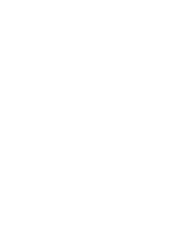6.123 × 100
=6.123
2.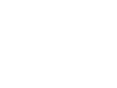3 × 7 × 11 × 13 = 3003 + 1
=3004
3. √144 = 12cm → one side
Perimeter = 12 × 4 = 48m
4. 2x2 − x − 6
P = −12     S = −1  F = −4,3
2x2 − 4x + 3x − 6
2x(x −2) +3(x−2)
(2x + 3)(x −2)
(2x+3)(x−2) = 0
x = −1½ or 2
5. 3x − 2 = 5x+8
−2x = 10
x ≥ −5
5x + 8 ≤ 3x+15
2x ≤ 7
x = 3½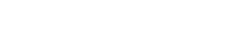Integral values
= -4, -3, -2,-1,0, 1, 2, 3
6. a= v−u
t
5 = 60 − u
5
25 = 60−u
u = 60−25
initial velocity = 35m/s   (check units)
7.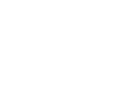NB Tr is a resultant vector (check the two arrows)
8.
1.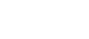2.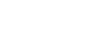9. 9x + 32x = 54
32x + 32x = 54
let 32x = y
2y = 54
y = 27
32x = 33
2x = 3
x =1½
10. Sum of interior angles
180(n−2) = 3240
180           180
n − 2 = 18
n = 20 sides
exterior = 360 =18°
20
11.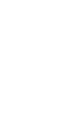1728 = 26 × 33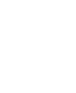1936 = 24 × 112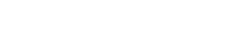= 3/11
12.   16     = 16 ×   1
2.674             2.674
0.3739 ×16
= 5.984
24        24 ×    1
0.1369            0.1369
7.163 × 24
=171.912
5.983 + 171.912
=177.896
13. Numerator
5/2 − 2/3 = 15 − 4 = 11/6
6
½ of 7/27/4     3/2 × 11/
7/411/418/4 = 4½
Denominator
¾ of 5/215/8 × 2/115/
9/2 × 4/156/5
=11/5
14. 2x + 3y =1
2x = 1 − 3y
2
x ( 1 − 3y
2
3 ( 1 − 3y ) − 2y = 8
2
3(1−3y) −4y = 16
3 − 9y − 4y =16
−13y =13
y = −1
x = 1 − (3x −1) = 1+3 = 4 = 2
2                2      2
15. 3y + 2x = 9
3y = −2x + 9
y = −2x + 3
3
Gradients are equal to (parallel lines)
= −2/3
a − 7−2 (cross multiply)
13 − a      3
3(a−7) = −2(13−a)
3a − 21 = −26 + 2a
a = −5
16.
1. ASF = 16:25
LSF = √16:√25
= 4:5
smaller:bigger
2. 4/528/x (cross multiply)
4x = 5 × 28
4          4
x = 35cm diameter
17.
1.  marks F x fx 199-203 204-208 209-213  214-218 219-223 224-228 229-233 234-238 239-243 244-248249 - 253 5 2 3 2 2 7 1 0 3 1 4 201  206 211 216 221 226 231 236 241 246 251 1005  412 633 432 442 1582 231   0 723 246 1004
f=30        Σfx=6710
2. Modal class = 224-228
3. Mean = Σfx = 6710 = 223.67
Σf       30
4. 30th = 15th + 16th = 15.5th
2                 2
223.5 + (15.5 −14 × 5)
7
223.5 + (1.5 × 5)
7
= 224.57marks
18.
1.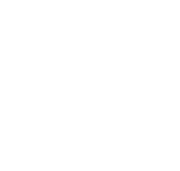2.  60 − 30 = 30 = 5m/s2 (check units)
6          6
3. Total distance = area under the graph
½ × 90 × 6 = 270m
5 × 60 = 300m
½ × 3 × 60 = 90m
270+300+90=660m
19.
1.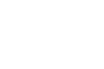sin−1 (6.5) = sin−1 0.8125
8             54.34  × 2
<XO1Y = 108.68°
2. sin−1 6.5/10   sin−1 0.65 = 40.54 × 2
<XO2Y  = 81.082
3. πrθ/360
3.142 × 8 ×8 = 108.68 = 60.71cm2
360
4. 3.142 × 10 × 10 = 81.08
360
= 70.765cm2
5. ½ × 8 × 8 sin 108.68
60.71 − 30.31cm2
= 30.4cm2
½ × 10 × 10 sin 81.08
= 49.4cm2
70.765 − 49.4
=21.365cm2
30.4 + 21.365
=51.765cm2
20.
1.
1. → = → + →
AB   AO+OB
= −a + b
= b − a
2. →  = → +→
OM   OA  AM
= a2/5 (b − a)
=a2/5b − 2/5a
=3/5a2/5b
3. →      →      →
BK = BO + OK
=−b + ½a
2.
1.         →       →
PQ = OQ − OP
=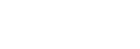2. 6 + 8  , 10+14
2           2
(7, 12)
3.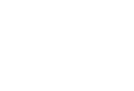=√337
=18.36units
21.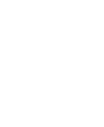25 = x + 15
10       x
25x = 10x + 150
15x = 150
x = 10cm
1. 10 + 15 = 25cm
2. Volume of bigger cone
1/3 × 3.142 × 25 × 25 × 25
=16364.58cm3
Volume of smaller cone
1/3 × 3.142 × 10 × 10 × 10
=1047.33cm3
frustrum = 16364.58 − 1047.33
= 15.317.25litres
3. Slant height (L) = √(252 + 252)
=35.36cm
(l) = 102 + 102
=14.142cm
Curved area
3.142 × 25 × 35.36  = 2777.53cm2
3.142 × 10 × 14.142 = 444.34cm2
2333.19cm2
3.142 × 10 × 10 = 314.2cm2
3.142 × 25 × 25 = 1963.75cm2
Total  = 4611.14cm2
22.
1. 3x + 4y = 12
4y = −3x + 12
y = −¾x + 3
2. Cuts the x=axis at y=0
3x + 4y = 12
3x = 12
x = 4
P(4,0)
Cuts the y-axis at x=0
4y = 12
y = 3
Q(0,3)
3. Gradient of L2= ¾x = 1
x = 4/3
y−5 = 4   (cross multiply)
x+4    3
3y − 15 = 4x + 16
3y − 4x = 31
3y = 4x + 31
y = 4/3x + 31/3
23. L1
(−2,0)(0,4)
4 − 0 = 4 = 2
0−−2    2
y     = 2
x + 2
y = 2x + 4
y − 2x = 4
1 − 4 = − 3 ≤ 4
y − 2x ≤ 4
L2
(0,2)(2,0)
0 − 2 = −2 = −1
2−0       2
y−2 = −1
x
y − 2 = −x
x+y = 2
3 > 2
x+y > 2
L3
(0,4)(4,0)
0 − 4 = −4 = −1
4−0       4
y−4 = −1
x
y − 4 = −x
x+y = 4
3 ≤ 4
x+y ≤ 4
L4
y = 4
y ≤ 4
1 ≤ 4
24.
1. Distance = 72 + 78
= 150m
Relative speed = 108 + 72 = 180km/hr
= 10/36 × 180 = 50m/s
T = D/S150/50 = 3seconds
2. D = 120 × 8/3 = 320km
320 = 80 litres × 64 = Shs 5120
4
3. ½ × 16km/hr = 8km
40 − 8 = 32km
32   = 32 = 1hr 20minutes
16+8    24
8:00
+1:20
9:20am

• ✔ To read offline at any time.
• ✔ To Print at your convenience
• ✔ Share Easily with Friends / Students